<input id="0qass"><u id="0qass"></u></input>
• <input id="0qass"><u id="0qass"></u></input>

# 梯度下降算法原理講解——機器學習

### 博文目錄

#### 1. 概述

本文將從一個下山的場景開始，先提出梯度下降算法的基本思想，進而從數學上解釋梯度下降算法的原理，解釋為什么要用梯度，最后實現一個簡單的梯度下降算法的實例！

#### 2. 梯度下降算法

##### 2.1 場景假設

梯度下降法的基本思想可以類比為一個下山的過程。
假設這樣一個場景：一個人被困在山上，需要從山上下來(找到山的最低點，也就是山谷)。但此時山上的濃霧很大，導致可視度很低；因此，下山的路徑就無法確定，必須利用自己周圍的信息一步一步地找到下山的路。這個時候，便可利用梯度下降算法來幫助自己下山。怎么做呢，首先以他當前的所處的位置為基準，尋找這個位置最陡峭的地方，然后朝著下降方向走一步，然后又繼續以當前位置為基準，再找最陡峭的地方，再走直到最后到達最低處；同理上山也是如此，只是這時候就變成梯度上升算法了##### 2.2 梯度下降

梯度下降的基本過程就和下山的場景很類似。

首先，我們有一個可微分的函數。這個函數就代表著一座山。我們的目標就是找到這個函數的最小值，也就是山底。根據之前的場景假設，最快的下山的方式就是找到當前位置最陡峭的方向，然后沿著此方向向下走，對應到函數中，就是找到給定點的梯度 ，然后朝著梯度相反的方向，就能讓函數值下降的最快！因為梯度的方向就是函數之變化最快的方向(在后面會詳細解釋)
所以，我們重復利用這個方法，反復求取梯度，最后就能到達局部的最小值，這就類似于我們下山的過程。而求取梯度就確定了最陡峭的方向，也就是場景中測量方向的手段。那么為什么梯度的方向就是最陡峭的方向呢？接下來，我們從微分開始講起：

###### 2.2.1 微分

看待微分的意義，可以有不同的角度，最常用的兩種是：

• 函數圖像中，某點的切線的斜率
• 函數的變化率
幾個微分的例子：

1.單變量的微分，函數只有一個變量時

d ( x 2 ) d x = 2 x \frac{d(x^2)}{dx}=2x

d ( ? 2 y 5 ) d y = ? 10 y 4 \frac{d(-2y^5)}{dy}=-10y^4

d ( 5 ? θ ) 2 d θ = ? 2 ( 5 ? θ ) \frac{d(5-\theta )^2}{d\theta}=-2(5-\theta)

2.多變量的微分，當函數有多個變量的時候，即分別對每個變量進行求微分

? ? x ( x 2 y 2 ) = 2 x y 2 \frac{\partial}{\partial x}(x^2y^2) = 2xy^2

? ? y ( ? 2 y 5 + z 2 ) = ? 10 y 4 \frac{\partial}{\partial y}(-2y^5+z^2) = -10y^4

? ? θ 2 ( 5 θ 1 + 2 θ 2 ? 12 θ 3 ) = 2 \frac{\partial}{\partial \theta_{2}}(5\theta_{1} + 2\theta_{2} - 12\theta_{3}) = 2

? ? θ 2 ( 0.55 ? ( 5 θ 1 + 2 θ 2 ? 12 θ 3 ) ) = ? 2 \frac{\partial}{\partial \theta_{2}}(0.55 - (5\theta_{1} + 2\theta_{2} - 12\theta_{3})) = -2

###### 2.2.2 梯度

梯度實際上就是多變量微分的一般化。
下面這個例子：

J ( Θ ) = 0.55 ? ( 5 θ 1 + 2 θ 2 ? 12 θ 3 ) J(\Theta ) = 0.55 - (5\theta_{1} + 2\theta_{2} - 12\theta_{3})

▽ J ( Θ ) = < ? J ? θ 1 , ? J ? θ 2 , ? J ? θ 3 > = ( ? 5 , ? 2 , 12 ) \triangledown J(\Theta ) = \left < \frac{\partial J}{\partial \theta_{1}}, \frac{\partial J}{\partial \theta_{2}},\frac{\partial J}{\partial \theta_{3}} \right > =(-5,-2,12)

我們可以看到，梯度就是分別對每個變量進行微分，然后用逗號分割開，梯度是用<>包括起來，說明梯度其實一個向量。

梯度是微積分中一個很重要的概念，之前提到過梯度的意義

• 在單變量的函數中，梯度其實就是函數的微分，代表著函數在某個給定點的切線的斜率
• 在多變量函數中，梯度是一個向量，向量有方向，梯度的方向就指出了函數在給定點的上升最快的方向

這也就說明了為什么我們需要千方百計的求取梯度！我們需要到達山底，就需要在每一步觀測到此時最陡峭的地方，梯度就恰巧告訴了我們這個方向。梯度的方向是函數在給定點上升最快的方向，那么梯度的反方向就是函數在給定點下降最快的方向，這正是我們所需要的。所以我們只要沿著梯度的方向一直走，就能走到局部的最低點！

##### 2.3 數學解釋

首先給出數學公式：

Θ 1 = Θ 0 + α ▽ J ( Θ ) → e v a l u a t e d a t Θ 0 {\color{Red} \Theta^1} = {\color{Blue} \Theta^0} + {\color{Green} \alpha} {\color{Purple} \triangledown J(\Theta)}\rightarrow evaluated at \Theta^0

此公式的意義是：J是關于Θ的一個函數，我們當前所處的位置為Θ0點，要從這個點走到J的最小值點，也就是山底。首先我們先確定前進的方向，也就是梯度的反向，然后走一段距離的步長，也就是α，走完這個段步長，就到達了Θ1這個點！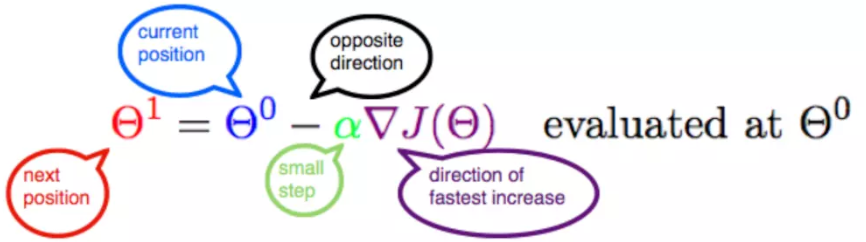###### 2.3.1 α

α在梯度下降算法中被稱作為學習率或者步長，意味著我們可以通過α來控制每一步走的距離，以保證不要步子跨的太大扯著蛋，哈哈，其實就是不要走太快，錯過了最低點。同時也要保證不要走的太慢，導致太陽下山了，還沒有走到山下。所以α的選擇在梯度下降法中往往是很重要的！α不能太大也不能太小，太小的話，可能導致遲遲走不到最低點，太大的話，會導致錯過最低點！

###### 2.3.2 梯度要乘以一個負號

梯度前加一個負號，就意味著朝著梯度相反的方向前進！我們在前文提到，梯度的方向實際就是函數在此點上升最快的方向！而我們需要朝著下降最快的方向走，自然就是負的梯度的方向，所以此處需要加上負號；那么如果時上坡，也就是梯度上升算法，當然就不需要添加負號了。

#### 3. 實例

我們已經基本了解了梯度下降算法的計算過程，那么我們就來看幾個梯度下降算法的小實例，首先從單變量的函數開始，然后介紹多變量的函數。

##### 3.1 單變量函數的梯度下降

我們假設有一個單變量的函數

J ( θ ) = θ 2 J(\theta) = \theta^2

函數的微分，直接求導就可以得到

J ′ ( θ ) = 2 θ J'(\theta) = 2\theta

初始化，也就是起點，起點可以隨意的設置，這里設置為1

θ 0 = 1 \theta^0 = 1

學習率也可以隨意的設置，這里設置為0.4

α = 0.4 \alpha = 0.4

根據梯度下降的計算公式

Θ 1 = Θ 0 + α ▽ J ( Θ ) → e v a l u a t e d a t Θ 0 {\color{Red} \Theta^1} = {\color{Blue} \Theta^0} + {\color{Green} \alpha} {\color{Purple} \triangledown J(\Theta)}\rightarrow evaluated at \Theta^0

我們開始進行梯度下降的迭代計算過程：

θ 0 = 1 \theta^0 = 1

θ 1 = θ 0 ? α ? J ′ ( θ 0 ) = 1 ? 0.4 ? 2 = 0.2 \theta^1 = \theta^0 - \alpha*J'(\theta^0)=1 - 0.4*2 = 0.2

θ 2 = θ 1 ? α ? J ′ ( θ 1 ) = 0.2 ? 0.4 ? 0.4 = 0.04 \theta^2 = \theta^1 - \alpha*J'(\theta^1)= 0.2 - 0.4*0.4=0.04

θ 3 = 0.008 \theta^3 = 0.008

θ 4 = 0.0016 \theta^4 = 0.0016

如圖，經過四次的運算，也就是走了四步，基本就抵達了函數的最低點，也就是山底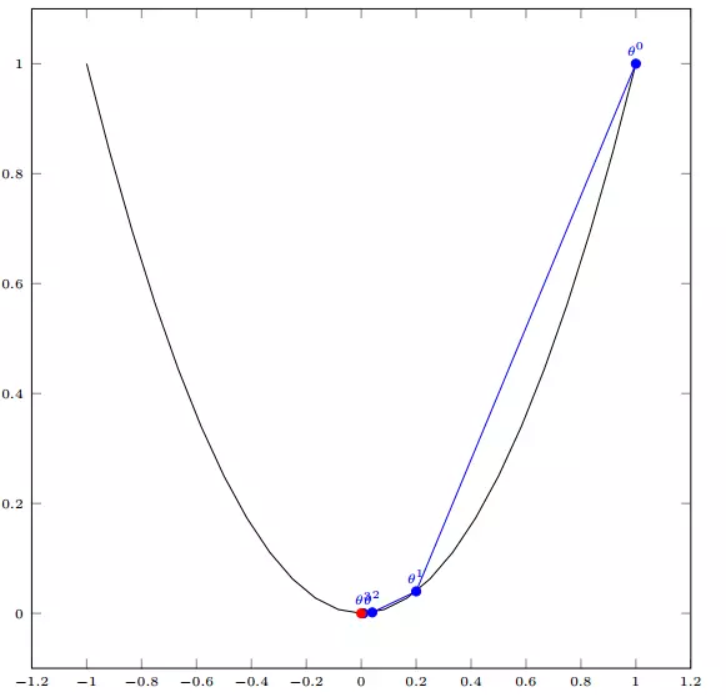##### 3.2 多變量函數的梯度下降

我們假設有一個目標函數

J ( Θ ) = θ 1 2 + θ 2 2 J(\Theta) = \theta_{1}^2 + \theta_{2}^2

現在要通過梯度下降法計算這個函數的最小值。我們通過觀察就能發現最小值其實就是 (0，0)點。但是接下來，我們會從梯度下降算法開始一步步計算到這個最小值！
我們假設初始的起點為：

Θ 0 = ( 1 , 3 ) \Theta^0 = (1, 3)

初始的學習率為：

α = 0.1 \alpha = 0.1

函數的梯度為：

▽ J ( Θ ) = < 2 θ 1 , 2 θ 2 > \triangledown J(\Theta ) = \left < 2\theta_{1},2\theta_{2} \right >

進行多次迭代：

Θ 0 = ( 1 , 3 ) \Theta^0 = (1, 3)

Θ 1 = Θ 0 ? α ▽ J ( Θ ) = ( 1 , 3 ) ? 0.1 ? ( 2 , 6 ) = ( 0.8 , 2.4 ) \Theta^1 = \Theta^0 - \alpha\triangledown J(\Theta ) = (1,3) - 0.1*(2, 6)=(0.8, 2.4)

Θ 2 = ( 0.8 , 2.4 ) ? 0.1 ? ( 1.6 , 4.8 ) = ( 0.64 , 1.92 ) \Theta^2 = (0.8, 2.4) - 0.1*(1.6, 4.8)=(0.64, 1.92)

Θ 3 = ( 0.5124 , 1.536 ) \Theta^3 =(0.5124, 1.536)

Θ 4 = ( 0.4096 , 1.228800000000001 ) \Theta^4 =(0.4096, 1.228800000000001)
? \vdots
Θ 10 = ( 0.1073741824000003 , 0.32212254720000005 ) \Theta^{10} =(0.1073741824000003, 0.32212254720000005)
? \vdots
Θ 50 = ( 1.141798154164342 e ? 05 , 3.42539442494306 e ? 05 ) \Theta^{50} =(1.141798154164342e^{-05}, 3.42539442494306e^{-05})
? \vdots
Θ 100 = ( 1.6296287810675902 e ? 10 , 4.8888886343202771 e ? 10 ) \Theta^{100} =(1.6296287810675902e^{-10}, 4.8888886343202771e^{-10})

我們發現，已經基本靠近函數的最小值點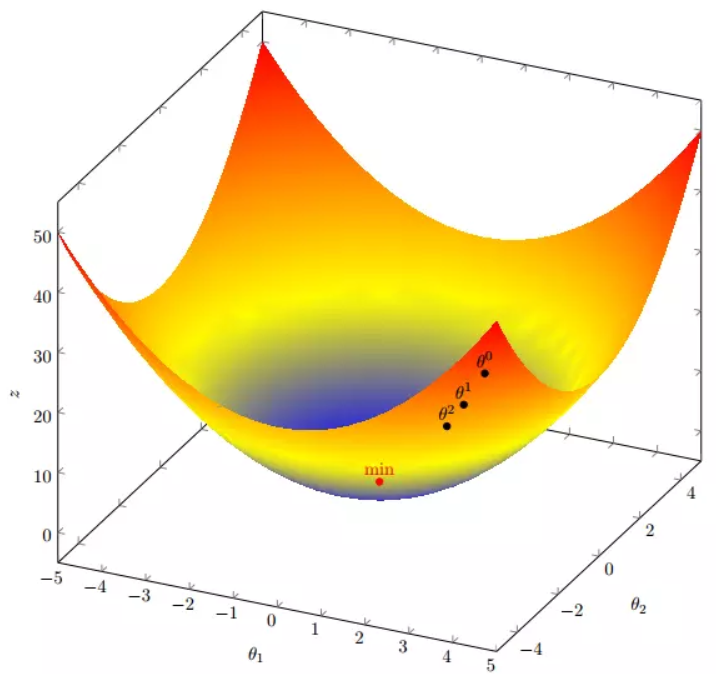#### 4. 代碼實現

##### 4. 1 場景分析

下面我們將用python實現一個簡單的梯度下降算法。場景是一個簡單的線性回歸的例子：假設現在我們有一系列的點，如下圖所示：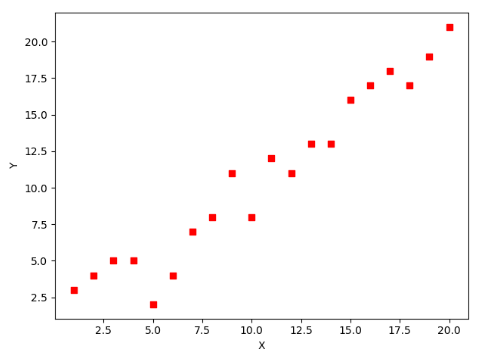我們將用梯度下降法來擬合出這條直線！

首先，我們需要定義一個代價函數，在此我們選用均方誤差代價函數（也稱平方誤差代價函數）

J ( Θ ) = 1 2 m ∑ i = 1 m ( h θ ( x ( i ) ) ? y ( i ) ) 2 J(\Theta) = \frac{1}{2m}\sum_{i=1}^{m}(h_{\theta}(x^{(i)})-y^{(i)})^2

此公式中

• m是數據集中數據點的個數，也就是樣本數
• ?是一個常量，這樣是為了在求梯度的時候，二次方乘下來的2就和這里的?抵消了，自然就沒有多余的常數系數，方便后續的計算，同時對結果不會有影響
• y 是數據集中每個點的真實y坐標的值，也就是類標簽
• h 是我們的預測函數（假設函數），根據每一個輸入x，根據Θ 計算得到預測的y值，即

h Θ ( x ( i ) ) = Θ 0 + Θ 1 x 1 ( i ) h_{\Theta}(x^{(i)}) = \Theta_{0} + \Theta_{1}x_{1}^{(i)}

我們可以根據代價函數看到，代價函數中的變量有兩個，所以是一個多變量的梯度下降問題，求解出代價函數的梯度，也就是分別對兩個變量進行微分

▽ J ( Θ ) = < δ J δ Θ 0 , δ J δ Θ 1 > \triangledown J(\Theta ) = \left < \frac{\delta J}{\delta \Theta_{0}}, \frac{\delta J}{\delta \Theta_{1}} \right >

δ J δ Θ 0 = 1 m ∑ i = 1 m ( h Θ ( x ( i ) ) ? y ( i ) ) \frac{\delta J}{\delta \Theta_{0}} = \frac{1}{m}\sum_{i=1}^{m}(h_{\Theta}(x^{(i)})-y^{(i)})

δ J δ Θ 1 = 1 m ∑ i = 1 m ( h Θ ( x ( i ) ) ? y ( i ) ) x 1 ( i ) \frac{\delta J}{\delta \Theta_{1}} = \frac{1}{m}\sum_{i=1}^{m}(h_{\Theta}(x^{(i)})-y^{(i)})x_{1}^{(i)}

明確了代價函數和梯度，以及預測的函數形式。我們就可以開始編寫代碼了。但在這之前，需要說明一點，就是為了方便代碼的編寫，我們會將所有的公式都轉換為矩陣的形式，python中計算矩陣是非常方便的，同時代碼也會變得非常的簡潔。
為了轉換為矩陣的計算，我們觀察到預測函數的形式

h Θ ( x ( i ) ) = Θ 0 + Θ 1 x ( i ) h_{\Theta}(x^{(i)}) = \Theta_{0} + \Theta_{1}x^{(i)}

我們有兩個變量，為了對這個公式進行矩陣化，我們可以給每一個點x增加一維，這一維的值固定為1，這一維將會乘到Θ0上。這樣就方便我們統一矩陣化的計算

( x 1 ( i ) , y ( i ) ) → ( x 0 ( i ) , x 1 ( i ) , y ( i ) ) w i t h x 0 ( i ) = 1 ? i (x_{1}^{(i)},y^{(i)})\rightarrow (x_{0}^{(i)},x_{1}^{(i)},y^{(i)}) with x_{0}^{(i)} = 1 \forall _{i}

然后我們將代價函數和梯度轉化為矩陣向量相乘的形式

J ( Θ ) = 1 2 m ( X Θ ? y ? ) T ( X Θ ? y ? ) J(\Theta) = \frac{1}{2m}(X\Theta - \vec{y})^{T}(X\Theta - \vec{y})

▽ J ( Θ ) = 1 m X T ( X Θ ? y ? ) ) \triangledown J(\Theta) = \frac{1}{m}X^{T}(X\Theta - \vec{y}))

##### 4. 2 代碼

首先，我們需要定義數據集和學習率

#!/usr/bin/env python3
# -*- coding: utf-8 -*-
# @Time    : 2019/1/21 21:06
# @Author  : Arrow and Bullet
# @Software: PyCharm

from numpy import *

# 數據集大小 即20個數據點
m = 20
# x的坐標以及對應的矩陣
X0 = ones((m, 1))  # 生成一個m行1列的向量，也就是x0，全是1
X1 = arange(1, m+1).reshape(m, 1)  # 生成一個m行1列的向量，也就是x1，從1到m
X = hstack((X0, X1))  # 按照列堆疊形成數組，其實就是樣本數據
# 對應的y坐標
y = np.array([
3, 4, 5, 5, 2, 4, 7, 8, 11, 8, 12,
11, 13, 13, 16, 17, 18, 17, 19, 21
]).reshape(m, 1)
# 學習率
alpha = 0.01


接下來我們以矩陣向量的形式定義代價函數和代價函數的梯度

# 定義代價函數
def cost_function(theta, X, Y):
diff = dot(X, theta) - Y  # dot() 數組需要像矩陣那樣相乘，就需要用到dot()
return (1/(2*m)) * dot(diff.transpose(), diff)

# 定義代價函數對應的梯度函數
diff = dot(X, theta) - Y
return (1/m) * dot(X.transpose(), diff)


最后就是算法的核心部分，梯度下降迭代計算

# 梯度下降迭代
theta = array([1, 1]).reshape(2, 1)
theta = theta - alpha * gradient
return theta

print('optimal:', optimal)
print('cost function:', cost_function(optimal, X, Y))


當梯度小于1e-5時，說明已經進入了比較平滑的狀態，類似于山谷的狀態，這時候再繼續迭代效果也不大了，所以這個時候可以退出循環！
運行代碼，計算得到的結果如下：

print('optimal:', optimal)  # 結果 [[0.51583286][0.96992163]]
print('cost function:', cost_function(optimal, X, Y))  # 1.014962406233101


通過matplotlib畫出圖像，

# 根據數據畫出對應的圖像
def plot(X, Y, theta):
import matplotlib.pyplot as plt
ax = plt.subplot(111)  # 這是我改的
ax.scatter(X, Y, s=30, c="red", marker="s")
plt.xlabel("X")
plt.ylabel("Y")
x = arange(0, 21, 0.2)  # x的范圍
y = theta + theta*x
ax.plot(x, y)
plt.show()

plot(X1, Y, optimal)


所擬合出的直線如下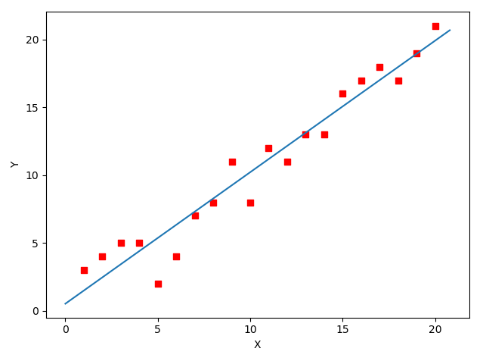全部代碼如下，大家有興趣的可以復制下來跑一下看一下結果：

#!/usr/bin/env python3
# -*- coding: utf-8 -*-
# @Time    : 2019/1/21 21:06
# @Author  : Arrow and Bullet
# @Software: PyCharm

from numpy import *

# 數據集大小 即20個數據點
m = 20
# x的坐標以及對應的矩陣
X0 = ones((m, 1))  # 生成一個m行1列的向量，也就是x0，全是1
X1 = arange(1, m+1).reshape(m, 1)  # 生成一個m行1列的向量，也就是x1，從1到m
X = hstack((X0, X1))  # 按照列堆疊形成數組，其實就是樣本數據
# 對應的y坐標
Y = array([
3, 4, 5, 5, 2, 4, 7, 8, 11, 8, 12,
11, 13, 13, 16, 17, 18, 17, 19, 21
]).reshape(m, 1)
# 學習率
alpha = 0.01

# 定義代價函數
def cost_function(theta, X, Y):
diff = dot(X, theta) - Y  # dot() 數組需要像矩陣那樣相乘，就需要用到dot()
return (1/(2*m)) * dot(diff.transpose(), diff)

# 定義代價函數對應的梯度函數
diff = dot(X, theta) - Y
return (1/m) * dot(X.transpose(), diff)

# 梯度下降迭代
theta = array([1, 1]).reshape(2, 1)
theta = theta - alpha * gradient
return theta

print('optimal:', optimal)
print('cost function:', cost_function(optimal, X, Y))

# 根據數據畫出對應的圖像
def plot(X, Y, theta):
import matplotlib.pyplot as plt
ax = plt.subplot(111)  # 這是我改的
ax.scatter(X, Y, s=30, c="red", marker="s")
plt.xlabel("X")
plt.ylabel("Y")
x = arange(0, 21, 0.2)  # x的范圍
y = theta + theta*x
ax.plot(x, y)
plt.show()

plot(X1, Y, optimal)


#### 5. 小結

至此，就基本介紹完了梯度下降法的基本思想和算法流程，并且用python實現了一個簡單的梯度下降算法擬合直線的案例！
最后，我們回到文章開頭所提出的場景假設:
這個下山的人實際上就代表了反向傳播算法，下山的路徑其實就代表著算法中一直在尋找的參數Θ，山上當前點的最陡峭的方向實際上就是代價函數在這一點的梯度方向，場景中觀測最陡峭方向所用的工具就是微分 。在下一次觀測之前的時間就是有我們算法中的學習率α所定義的。
可以看到場景假設和梯度下降算法很好的完成了對應！

本文部分內容來自一位前輩，非常感謝分享！謝謝！

已標記關鍵詞 清除標記插入表情還能輸入1000個字符
06-015萬+
11-032萬+
01-252071
04-143萬+
07-056萬+
03-037054
01-079062
02-265027
04-041348
10-212143
07-14
07-038185
08-16153
06-30465
07-192396
03-03611
12-114萬+
04-018194
??2020 CSDN 皮膚主題: 技術黑板 設計師:CSDN官方博客
多乐彩